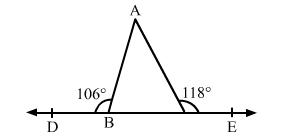# In the given figure, the side BC of ∆ ABC has been produced on both sides−on the left to D and on the right to E.

Question:

In the given figure, the side BC of ∆ ABC has been produced on both sides−on the left to D and on the right to E. If ∠ABD = 106° and ∠ACE = 118°, find the measure of each angle of the triangle.Solution:

Side BC of triangle ABC is produced to D.

$\therefore \angle A B C=\angle A+\angle C$

$\Rightarrow 106^{\circ}=\angle A+\angle C \quad \ldots(i)$

Also, side BC of triangle ABC is produced to E.

$\angle A C E=\angle A+\angle B$

$\Rightarrow 118^{\circ}=\angle A+\angle B \quad \ldots(i i)$

Adding $(i)$ and $(i i)$, we get:

$\angle A+\angle A+\angle B+\angle C=(106+118)^{\circ}$

$\Rightarrow(\angle A+\angle B+\angle C)+\angle A=224^{\circ} \quad\left[\because \angle A+\angle B+\angle C=180^{\circ}\right]$

$\Rightarrow 180^{\circ}+\angle A=224^{\circ}$

$\Rightarrow \angle A=44^{\circ}$

$\therefore \angle B=118^{\circ}-\angle A \quad[$ Using $(i i)]$

$\Rightarrow \angle B=(118-44)^{\circ}$

$\Rightarrow \angle B=74^{\circ}$

And,

$\angle C=106^{\circ}-\angle A \quad[$ Using $(i)]$

$\Rightarrow \angle C=(106-44)^{\circ}$

$\Rightarrow \angle C=62^{\circ}$